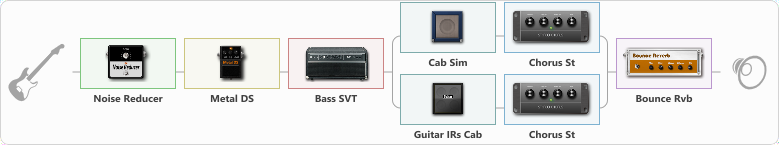# yrametal

Discussion in 'ToneLib-GFX presets' started by yrametal, May 7, 2021.

1. yrametal

Preset name: YRAMETAL

Effects chain:Effect: "Noise Reducer" (Dynamics / Filter), active - "yes"
{
"Sens" = 80
"Mode" = Hard
}

Effect: "Metal DS" (Overdrive / Distortion), active - "yes"
{
"Dist" = 60
"Bass" = 74
"Middle" = 60
"Treble" = 37
"Level" = 57
}

Effect: "Bass SVT" (Amp simulators), active - "yes"
{
"Bass Ctrl" = Cut
"Midrange" = 3 kHz
"Ultra Hi" = On
"Bass" = 1
"Middle" = 2
"Treble" = 1
"Volume" = 50
"Level (dB)" = -4
}

Effect: "Splitter" (Dynamics / Filter)
{
"A-Bypass" = Off
"A-Pan" = 0
"A-Level" = 55
"B-Bypass" = Off
"B-Pan" = 0
"B-Level" = 55

'A' branch:
{

Effect: "Cab Sim" (Cabinets), active - "yes"
{
"Model" = 4x12" Cali v30
"Level (dB)" = 3
}

Effect: "Chorus St" (Modulation / Sfx), active - "yes"
{
"Speed" = 1.9
"Depth" = 31
"Center" = 4.4
"Mix" = 68
}
}
'B' branch:
{

Effect: "Guitar IRs Cab" (Cabinets), active - "yes"
{
"Model" = Orange PPC (4x12")
"Mic Position" = Middle
"Mic Distance" = Middle
"Low Cut (Hz)" = 60
"Hi Cut (kHz)" = 20.0
"Mix" = 65
"Level (dB)" = 0
}

Effect: "Chorus St" (Modulation / Sfx), active - "yes"
{
"Speed" = 1.9
"Depth" = 31
"Center" = 4.4
"Mix" = 69
}
}
}

Effect: "Bounce Rvb" (Reverberation), active - "yes"
{
"Time" = 4.0
"PreDelay" = 0
"LoDamp" = 16
"HiDamp" = 26
"Mix" = 42
}

Note: You will need to download and install the ToneLib-GFX software to use the preset.

File size:
842 bytes
Views:
4,098